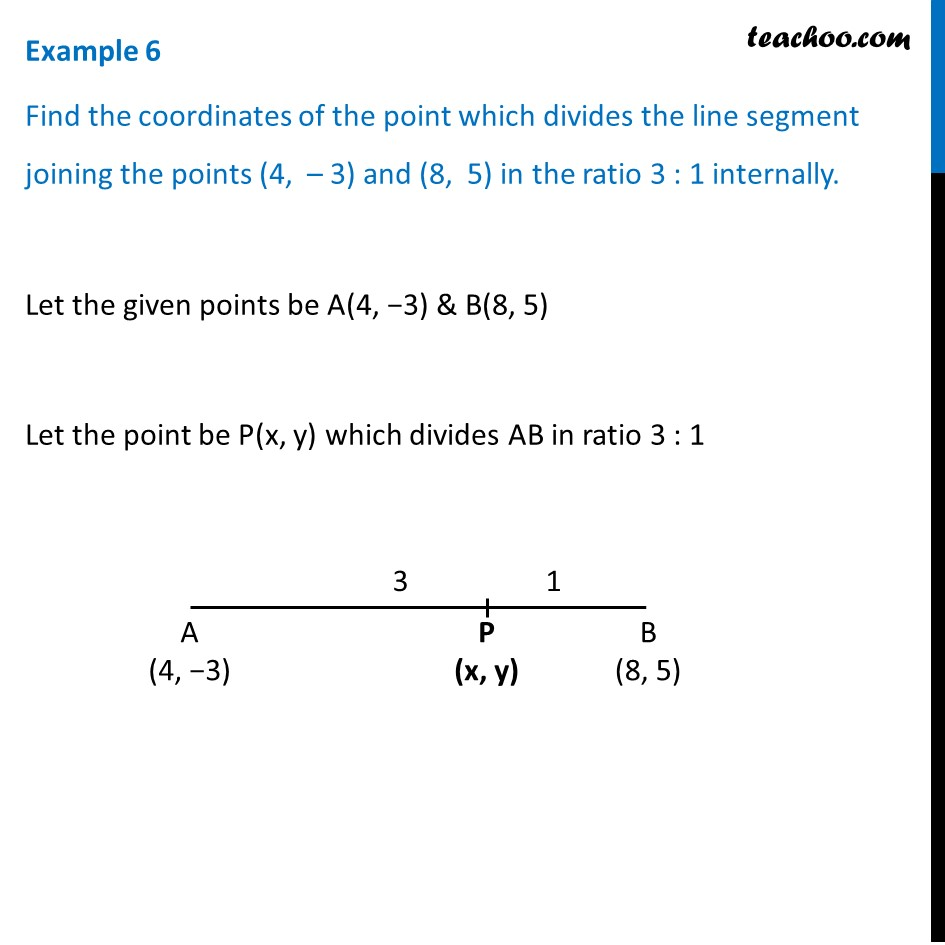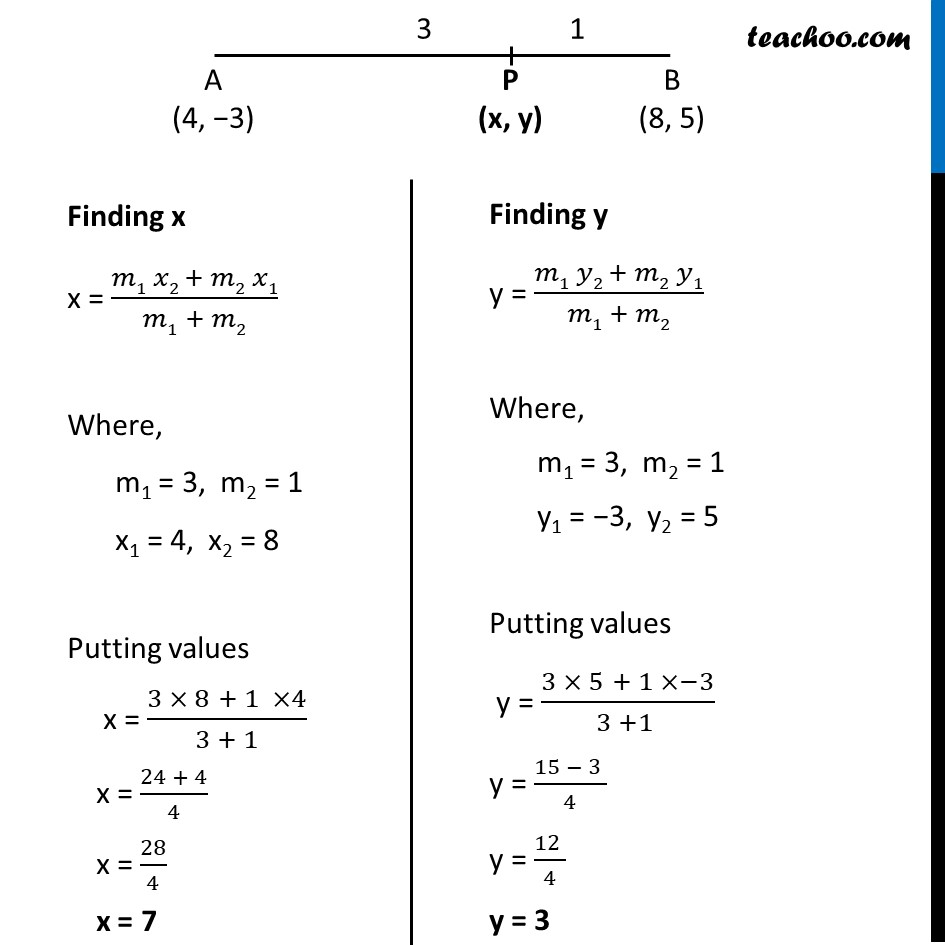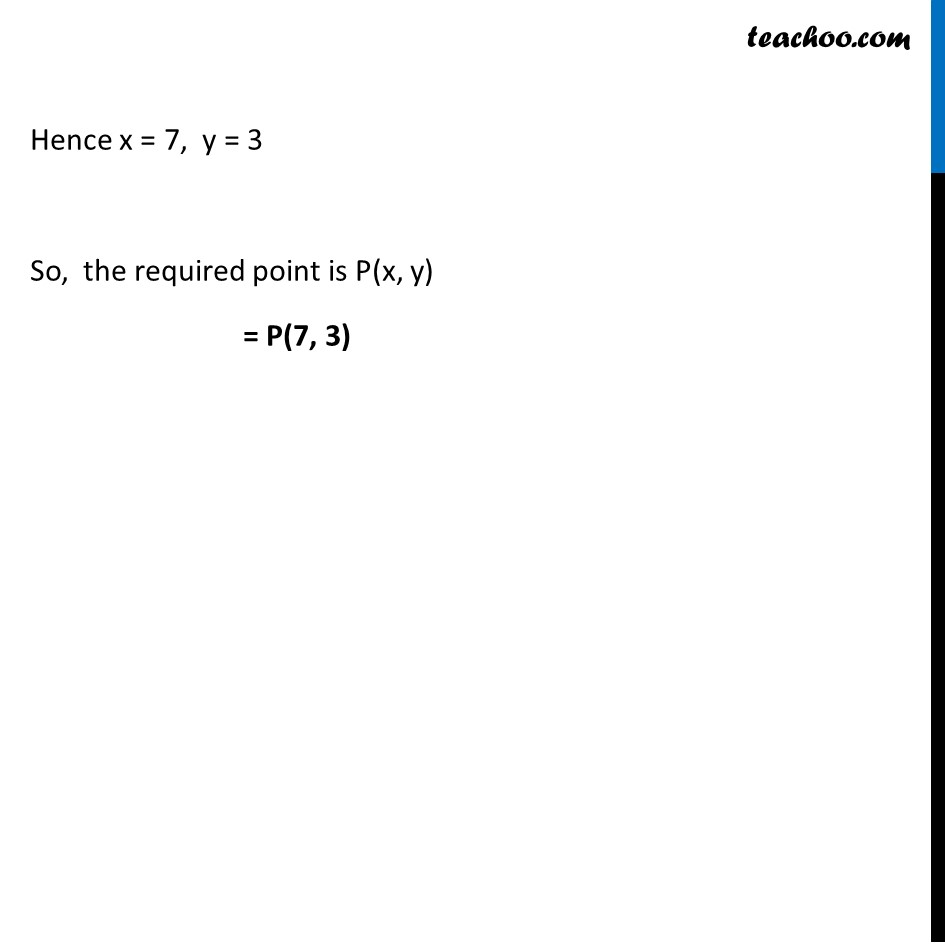Section Formula- Finding coordinates

Chapter 7 Class 10 Coordinate Geometry
Concept wiseIntroducing your new favourite teacher - Teachoo Black, at only ₹83 per month

### Transcript

Example 6 Find the coordinates of the point which divides the line segment joining the points (4, – 3) and (8, 5) in the ratio 3 : 1 internally. Let the given points be A(4, −3) & B(8, 5) Let the point be P(x, y) which divides AB in ratio 3 : 1 Finding x x = (𝑚1 𝑥2 + 𝑚2 𝑥1)/(𝑚1 + 𝑚2) Where, m1 = 3, m2 = 1 x1 = 4, x2 = 8 Putting values x = (3 × 8 + 1 ×4)/(3 + 1) x = (24 + 4)/4 x = 28/4 x = 7 Finding y y = (𝑚1 𝑦2 + 𝑚2 𝑦1)/(𝑚1 + 𝑚2) Where, m1 = 3, m2 = 1 y1 = −3, y2 = 5 Putting values y = (3 × 5 + 1 ×−3)/(3 +1 ) y = (15 − 3 )/4 y = (12 )/4 y = 3 Hence x = 7, y = 3 So, the required point is P(x, y) = P(7, 3)